# 10.6 Networks of uniform strings  (Page 3/3)

 Page 3 / 3

## Example #1: the tritar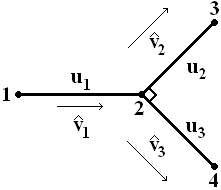The Tritar

Depicted above is a network of three strings called a tritar. We are interested in how the eigenvalues of this simple network vary with changes in the transverse stiffness k i of each string. We assume that the longitudinal stiffnesses σ i are 1 for each string, and we also assume that the lengths of the strings are all 1 for conveninence.

We define our orientation vectors by

$\begin{array}{c}\hfill \stackrel{^}{{v}_{1}}=\left[\begin{array}{c}1\\ 0\end{array}\right]\phantom{\rule{1.em}{0ex}}\phantom{\rule{1.em}{0ex}}\stackrel{^}{{v}_{2}}=\frac{1}{\sqrt{2}}\left[\begin{array}{c}1\\ 1\end{array}\right]\phantom{\rule{1.em}{0ex}}\phantom{\rule{1.em}{0ex}}\stackrel{^}{{v}_{3}}=\frac{1}{\sqrt{2}}\left[\begin{array}{c}1\\ -1\end{array}\right]\end{array}$

First, we compute the stiffness matrices. For the first string, we obtain

$\begin{array}{ccc}\hfill {P}_{1}& =& {K}_{1}\left(I-\stackrel{^}{{v}_{1}}{\stackrel{^}{{v}_{1}}}^{T}\right)+{\sigma }_{1}\stackrel{^}{{v}_{1}}{\stackrel{^}{{v}_{1}}}^{T}\hfill \\ & =& k\left(\left[\begin{array}{cc}1& 0\\ 0& 1\end{array}\right],-,\left[\begin{array}{cc}1& 0\\ 0& 0\end{array}\right]\right)+\left[\begin{array}{cc}1& 0\\ 0& 0\end{array}\right]\hfill \\ & =& \left[\begin{array}{cc}1& 0\\ 0& k\end{array}\right].\hfill \end{array}$

For the second string, we get

$\begin{array}{ccc}\hfill {P}_{2}& =& {K}_{2}\left(I-\stackrel{^}{{v}_{2}}{\stackrel{^}{{v}_{2}}}^{T}\right)+{\sigma }_{2}\stackrel{^}{{v}_{2}}{\stackrel{^}{{v}_{2}}}^{T}\hfill \\ & =& k\left(\left[\begin{array}{cc}1& 0\\ 0& 1\end{array}\right],-,\frac{1}{2},\left[\begin{array}{cc}1& 1\\ 1& 1\end{array}\right]\right)+\frac{1}{2}\left[\begin{array}{cc}1& 1\\ 1& 1\end{array}\right]\hfill \\ & =& \frac{1}{2}\left[\begin{array}{cc}k+1& -k+1\\ -k+1& k+1\end{array}\right],\hfill \end{array}$

and for string number three, we have

$\begin{array}{ccc}\hfill {P}_{3}& =& {K}_{3}\left(I-\stackrel{^}{{v}_{3}}{\stackrel{^}{{v}_{3}}}^{T}\right)+{\sigma }_{3}\stackrel{^}{{v}_{3}}{\stackrel{^}{{v}_{3}}}^{T}\hfill \\ & =& k\left(\left[\begin{array}{cc}1& 0\\ 0& 1\end{array}\right],-,\frac{1}{2},\left[\begin{array}{cc}1& -1\\ -1& 1\end{array}\right]\right)+\frac{1}{2}\left[\begin{array}{cc}1& -1\\ -1& 1\end{array}\right]\hfill \\ & =& \frac{1}{2}\left[\begin{array}{cc}k+1& k-1\\ k-1& k+1\end{array}\right].\hfill \end{array}$

Letting the vector ${u}_{i}={\left[{u}_{i1}\phantom{\rule{0.222222em}{0ex}}{u}_{i2}\right]}^{T}$ represent the displacements of string i , we obtain the following system of differential equations:

$\begin{array}{ccc}\hfill {P}_{1}{u}_{1}^{\text{'}\text{'}}& =& -{\lambda }^{2}{u}_{1}\hfill \\ \hfill {P}_{2}{u}_{2}^{\text{'}\text{'}}& =& -{\lambda }^{2}{u}_{2}\hfill \\ \hfill {P}_{3}{u}_{3}^{\text{'}\text{'}}& =& -{\lambda }^{2}{u}_{3}.\hfill \end{array}$

Expanding via matrix multiplication, we see that, component-wise, this translates into

$\begin{array}{ccc}\hfill {u}_{11}^{\text{'}\text{'}}& =& -{\lambda }^{2}{u}_{11}\hfill \\ \hfill k{u}_{12}^{\text{'}\text{'}}& =& -{\lambda }^{2}{u}_{12}\hfill \\ \hfill {s}_{2}^{\text{'}\text{'}}& =& -{\lambda }^{2}{s}_{2}\hfill \\ \hfill k{d}_{2}^{\text{'}\text{'}}& =& -{\lambda }^{2}{d}_{2}\hfill \\ \hfill k{s}_{3}^{\text{'}\text{'}}& =& -{\lambda }^{2}{s}_{3}\hfill \\ \hfill {d}_{3}^{\text{'}\text{'}}& =& -{\lambda }^{2}{d}_{3},\hfill \end{array}$

where

$\begin{array}{c}\hfill {s}_{i}={u}_{i1}+{u}_{i2}\\ \hfill {d}_{i}={u}_{i2}-{u}_{i2}.\end{array}$

for $i=1,2$ . For boundary conditions, we require that the ends be clamped:

$\begin{array}{ccc}\hfill {u}_{1}\left(0\right)& =& 0\hfill \\ \hfill {u}_{2}\left(1\right)& =& 0\hfill \\ \hfill {u}_{3}\left(1\right)& =& 0,\hfill \end{array}$

that there be continuity at the central node:

$\begin{array}{c}\hfill {u}_{1}\left(1\right)={u}_{2}\left(0\right)={u}_{3}\left(0\right),\end{array}$

and that the forces balance at the central node:

$\begin{array}{c}\hfill {P}_{1}{u}_{1}^{\text{'}}\left(1\right)-{P}_{2}{u}_{2}^{\text{'}}\left(0\right)-{P}_{3}{u}_{3}^{\text{'}}\left(0\right)=0.\end{array}$

As with many systems of differential equations, this one can be solved via the time-honored method of guessing. Noting that the differential equations of this form equate the second derivative of a function with a constant multiple of itself, wehypothesize that the solution for each component of displacement is some linear combination of sines and cosines:

$\begin{array}{ccc}\hfill {u}_{11}& =& {a}_{1,1}cos\lambda x+{b}_{1,1}sin\lambda x\hfill \\ \hfill {u}_{12}& =& {a}_{1,2}cos\frac{\lambda }{\sqrt{k}}x+{b}_{1,2}sin\frac{\lambda }{\sqrt{k}}x\hfill \\ \hfill {u}_{21}& =& {a}_{2}cos\lambda x+{b}_{2}sin\lambda x+{\alpha }_{2}cos\frac{\lambda }{\sqrt{k}}x+{\beta }_{2}sin\frac{\lambda }{\sqrt{k}}x\hfill \\ \hfill {u}_{22}& =& {a}_{2}cos\lambda x+{b}_{2}sin\lambda x-{\alpha }_{2}cos\frac{\lambda }{\sqrt{k}}x-{\beta }_{2}sin\frac{\lambda }{\sqrt{k}}x\hfill \\ \hfill {u}_{31}& =& {a}_{3}cos\frac{\lambda }{\sqrt{k}}x+{b}_{3}sin\frac{\lambda }{\sqrt{k}}x+{\alpha }_{3}cos\lambda x+{\beta }_{3}sin\lambda x\hfill \\ \hfill {u}_{32}& =& {a}_{3}cos\frac{\lambda }{\sqrt{k}}x+{b}_{3}sin\frac{\lambda }{\sqrt{k}}x-{\alpha }_{3}cos\lambda x-{\beta }_{3}sin\lambda x.\hfill \end{array}$

where we have guessed

$\begin{array}{ccc}\hfill {s}_{2}& =& 2{a}_{2}cos\lambda x+2{b}_{2}sin\lambda x\hfill \\ \hfill {d}_{2}& =& 2{\alpha }_{2}cos\frac{\lambda }{\sqrt{k}}x+2{\beta }_{2}sin\frac{\lambda }{\sqrt{k}}x\hfill \\ \hfill {s}_{3}& =& 2{a}_{3}cos\frac{\lambda }{\sqrt{k}}x+2{b}_{3}sin\frac{\lambda }{\sqrt{k}}x\hfill \\ \hfill {d}_{3}& =& 2{\alpha }_{3}cos\lambda x+2{\beta }_{3}sin\lambda x\hfill \end{array}$

and have used the above to translate these into u 21 , u 22 , u 31 , and u 32 . We need to determine the coefficients. Applying the boundary condition that ${u}_{1}\left(0\right)=0$ , we get

$\begin{array}{ccc}\hfill {a}_{1,1}& =& 0\hfill \\ \hfill {a}_{1,2}& =& 0.\hfill \end{array}$

The fact that ${u}_{2}\left(1\right)=0$ implies that

$\begin{array}{ccc}\hfill {a}_{2}cos\lambda +{b}_{2}sin\lambda & =& 0\hfill \\ \hfill {\alpha }_{2}cos\frac{\lambda }{\sqrt{k}}+{\beta }_{2}sin\frac{\lambda }{\sqrt{k}}& =& 0.\hfill \end{array}$

Likewise, for ${u}_{3}\left(1\right)=0$ , we get

$\begin{array}{ccc}\hfill {a}_{3}cos\frac{\lambda }{\sqrt{k}}+{b}_{3}sin\frac{\lambda }{\sqrt{k}}& =& 0\hfill \\ \hfill {\alpha }_{3}cos\lambda +{\beta }_{3}sin\lambda & =& 0.\hfill \end{array}$

Next, we apply the continuity conditions. Since ${u}_{1}\left(1\right)={u}_{2}\left(0\right)$ , we see that

$\begin{array}{ccc}\hfill {a}_{1,1}cos\lambda +{b}_{1,1}sin\lambda -{a}_{2}-{\alpha }_{2}& =& 0\hfill \\ \hfill {a}_{1,2}cos\frac{\lambda }{\sqrt{k}}+{b}_{1,2}sin\frac{\lambda }{\sqrt{k}}-{a}_{2}+{\alpha }_{2}& =& 0,\hfill \end{array}$

and because ${u}_{2}\left(0\right)={u}_{3}\left(0\right)$ we have

$\begin{array}{ccc}\hfill {a}_{2}-{a}_{3}& =& 0\hfill \\ \hfill {\alpha }_{2}-{\alpha }_{3}& =& 0.\hfill \end{array}$

Finally, from the force-balance equation, we have

$\begin{array}{ccc}\hfill -{a}_{1,1}\lambda sin\lambda & +& {b}_{1,1}\lambda cos\lambda -\frac{1}{2}\left(k+1\right)\left({b}_{2},\lambda ,+,{\beta }_{2},\frac{\lambda }{\sqrt{k}}\right)-\frac{1}{2}\left(k+1\right)\left({b}_{2},\lambda ,-,{\beta }_{2},\frac{\lambda }{\sqrt{k}}\right)-\frac{1}{2}\left(k-1\right)\left({b}_{3},\frac{\lambda }{\sqrt{k}},+,{\beta }_{3},\lambda \right)\hfill \\ & -& \frac{1}{2}\left(k+1\right)\left({b}_{3},\frac{\lambda }{\sqrt{k}},-,{\beta }_{3},\lambda \right)=0\hfill \end{array}$

and

$\begin{array}{ccc}\hfill -{a}_{1,2}\frac{\lambda }{\sqrt{k}}sin\frac{\lambda }{\sqrt{k}}& +& {b}_{1,2}\frac{\lambda }{\sqrt{k}}cos\frac{\lambda }{\sqrt{k}}-\frac{1}{2}\left(1-k\right)\left({b}_{2},\lambda ,+,{\beta }_{2},\frac{\lambda }{\sqrt{k}}\right)-\frac{1}{2}\left(k+1\right)\left({b}_{2},\lambda ,-,{\beta }_{2},\frac{\lambda }{\sqrt{k}}\right)-\frac{1}{2}\left(k-1\right)\left({b}_{3},\frac{\lambda }{\sqrt{k}},+,{\beta }_{3},\lambda \right)\hfill \\ & -& \frac{1}{2}k+1\left({b}_{3},\frac{\lambda }{\sqrt{k}},-,{\beta }_{3},\lambda \right)=0.\hfill \end{array}$

These twelve equations can be represented by a single matrix equation with the following coefficient matrix:

$\begin{array}{c}\hfill \left[\begin{array}{cccccccccccc}1& 0& 0& 0& 0& 0& 0& 0& 0& 0& 0& 0\\ 0& 1& 0& 0& 0& 0& 0& 0& 0& 0& 0& 0\\ 0& 0& 0& 0& cos\lambda & sin\lambda & 0& 0& 0& 0& 0& 0\\ 0& 0& 0& 0& 0& 0& cos\frac{\lambda }{\sqrt{k}}& sin\frac{\lambda }{\sqrt{k}}& 0& 0& 0& 0\\ 0& 0& 0& 0& 0& 0& 0& 0& cos\frac{\lambda }{\sqrt{k}}& sin\frac{\lambda }{\sqrt{k}}& 0& 0\\ 0& 0& 0& 0& 0& 0& 0& 0& 0& 0& cos\lambda & sin\lambda \\ cos\lambda & 0& sin\lambda & 0& -1& 0& -1& 0& 0& 0& 0& 0\\ 0& cos\frac{\lambda }{\sqrt{k}}& 0& sin\frac{\lambda }{\sqrt{k}}& -1& 0& 1& 0& 0& 0& 0& 0\\ 0& 0& 0& 0& 1& 0& 0& 0& -1& 0& 0& 0\\ 0& 0& 0& 0& 0& 0& 1& 0& 0& 0& -1& 0\\ -\lambda sin\lambda & 0& \lambda cos\lambda & 0& 0& -\lambda & 0& \frac{\lambda }{\sqrt{k}}& 0& \left(1-k\right)\frac{\lambda }{\sqrt{k}}& 0& -\lambda \\ 0& -\frac{\lambda }{\sqrt{k}}sin\frac{\lambda }{\sqrt{k}}& 0& \frac{\lambda }{\sqrt{k}}cos\frac{\lambda }{\sqrt{k}}& 0& -\lambda & 0& \frac{\lambda }{\sqrt{k}}& 0& -\frac{\lambda }{\sqrt{k}}& 0& \lambda \end{array}\right].\end{array}$

The determinant of this matrix is

$\begin{array}{ccc}& & -sin\left(\frac{\lambda }{\sqrt{k}}\right)sin\left(\lambda \right){l}^{2}\left(6,\phantom{\rule{0.166667em}{0ex}},cos,\left(\lambda \right),sin,\left(\frac{\lambda }{\sqrt{k}}\right),{k}^{2},cos,\left(\frac{\lambda }{\sqrt{k}}\right),sin,\left(\lambda \right),+,4,\phantom{\rule{0.166667em}{0ex}},{\left(cos,\left(\lambda \right)\right)}^{2},{\left(sin,\left(\frac{\lambda }{\sqrt{k}}\right)\right)}^{2},{k}^{3/2}\right)\hfill \\ & & \phantom{\rule{1.em}{0ex}}\left(+,3,\phantom{\rule{0.166667em}{0ex}},cos,\left(\lambda \right),sin,\left(\frac{\lambda }{\sqrt{k}}\right),k,cos,\left(\frac{\lambda }{\sqrt{k}}\right),sin,\left(\lambda \right),+,{\left(sin,\left(\lambda \right)\right)}^{2},{\left(cos,\left(\frac{\lambda }{\sqrt{k}}\right)\right)}^{2},{k}^{3/2},-,{\left(sin,\left(\lambda \right)\right)}^{2},{\left(cos,\left(\frac{\lambda }{\sqrt{k}}\right)\right)}^{2},\sqrt{k}\right)\hfill \\ & & \phantom{\rule{1.em}{0ex}}\left(+,2,\phantom{\rule{0.166667em}{0ex}},{\left(sin,\left(\lambda \right)\right)}^{2},{\left(cos,\left(\frac{\lambda }{\sqrt{k}}\right)\right)}^{2},{k}^{5/2}){k}^{-3/2},\hfill \end{array}$

and by substituting in the desired value of k and setting this determiniant to zero we can then solve for the eigenvalues λ of our tritar net. A plot of the first seven eigenvalues as a function of k is displayed below:First seven planar eigenvalues for a tritar net, computed with fzero().

As expected, the eigenvalues increase as k increases; however for eigenvalues beyond the first, we observe some rather strange behavior, which suggests that something has gone wrong in the above process. The eigenvalues in the above plot werecomputed using MATLAB's fzero() function at a tolerance of 1e-10. Using a more naive bisection method (which is less likely to lock onto the wrong root) at the same tolerance, we obtain the following plot: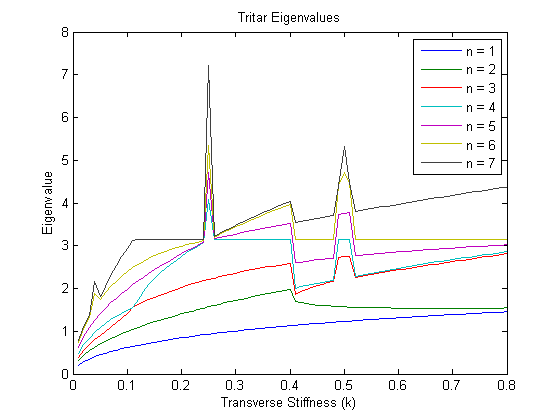First seven planar eigenvalues for a tritar net, computed with the bisection method.

This seems to have fixed some of the erratic behavior, but neither tightening the tolerance nor increasing the fine-ness of the mesh along which the determinant is evaluated provides much further improvement. On the other hand, it is apparent thatthe solver's structure and parameters impacts the shape of the plots. Perhaps a better solver of some sort (e.g. Newton's method, but adapted to search only in a given interval) can fix more of the problem.

## Example #2: the quintar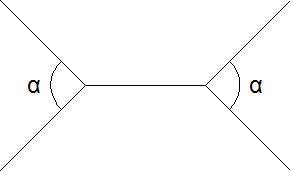A depiction of the quintar, with the end angles at 90 degrees.

Rather than develop all of the mathematical relations as in the previous example, it should suffice to say that the same procedure is followed. The solutions to the differential equations are still sums of sines and cosines, but more equations have been added to the system. The function obtained by setting the determinant equal to zero is not enlightening and longer than that for the quintar, and so only the final plots will be presented here. By iteratively increasing the angle at the ends of the network, a plot of the angle versus the eigenvalues is obtained in which traces are formed as the eigenvalues change. It is interesting to note how some of the eigenvalues increase in magnitude while others decrease.

A second plot is presented in which the first nine eigenvalues are plotted versus the transverse stiffness parameter for our analytic model. A region of stiffnesses was chosen where the root-finding algorithm is able to successfully lock onto the zeroes of the determinant as they change. It is clear from this plot that for this range of stiffnesses, increasing stiffness results in the modes of vibration increasing in frequency. This result is expected, since in general stiffer members possess higher vibrational frequencies.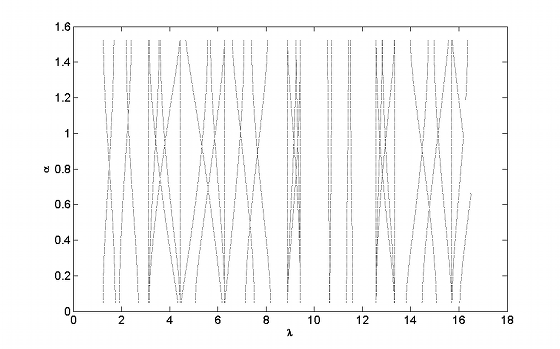A plot of the opening angle at the ends versus the eigenvalues.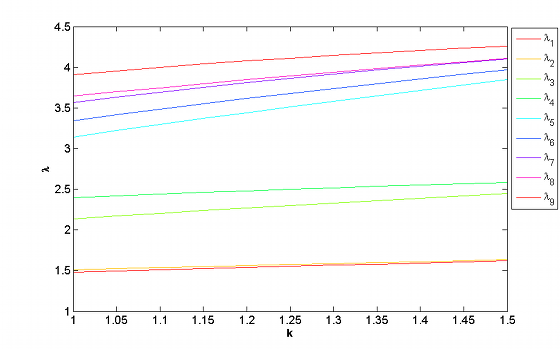A plot of the first nine eigenvalues for planar modes versus the transverse stiffness.

#### Questions & Answers

Is there any normative that regulates the use of silver nanoparticles?
Damian Reply
what king of growth are you checking .?
Renato
What fields keep nano created devices from performing or assimulating ? Magnetic fields ? Are do they assimilate ?
Stoney Reply
why we need to study biomolecules, molecular biology in nanotechnology?
Adin Reply
?
Kyle
yes I'm doing my masters in nanotechnology, we are being studying all these domains as well..
Adin
why?
Adin
what school?
Kyle
biomolecules are e building blocks of every organics and inorganic materials.
Joe
anyone know any internet site where one can find nanotechnology papers?
Damian Reply
research.net
kanaga
sciencedirect big data base
Ernesto
Introduction about quantum dots in nanotechnology
Praveena Reply
what does nano mean?
Anassong Reply
nano basically means 10^(-9). nanometer is a unit to measure length.
Bharti
do you think it's worthwhile in the long term to study the effects and possibilities of nanotechnology on viral treatment?
Damian Reply
absolutely yes
Daniel
how to know photocatalytic properties of tio2 nanoparticles...what to do now
Akash Reply
it is a goid question and i want to know the answer as well
Maciej
characteristics of micro business
Abigail
for teaching engĺish at school how nano technology help us
Anassong
Do somebody tell me a best nano engineering book for beginners?
s. Reply
there is no specific books for beginners but there is book called principle of nanotechnology
NANO
what is fullerene does it is used to make bukky balls
Devang Reply
are you nano engineer ?
s.
fullerene is a bucky ball aka Carbon 60 molecule. It was name by the architect Fuller. He design the geodesic dome. it resembles a soccer ball.
Tarell
what is the actual application of fullerenes nowadays?
Damian
That is a great question Damian. best way to answer that question is to Google it. there are hundreds of applications for buck minister fullerenes, from medical to aerospace. you can also find plenty of research papers that will give you great detail on the potential applications of fullerenes.
Tarell
what is the Synthesis, properties,and applications of carbon nano chemistry
Abhijith Reply
Mostly, they use nano carbon for electronics and for materials to be strengthened.
Virgil
is Bucky paper clear?
CYNTHIA
carbon nanotubes has various application in fuel cells membrane, current research on cancer drug,and in electronics MEMS and NEMS etc
NANO
so some one know about replacing silicon atom with phosphorous in semiconductors device?
s. Reply
Yeah, it is a pain to say the least. You basically have to heat the substarte up to around 1000 degrees celcius then pass phosphene gas over top of it, which is explosive and toxic by the way, under very low pressure.
Harper
Do you know which machine is used to that process?
s.
how to fabricate graphene ink ?
SUYASH Reply
for screen printed electrodes ?
SUYASH
What is lattice structure?
s. Reply
of graphene you mean?
Ebrahim
or in general
Ebrahim
in general
s.
Graphene has a hexagonal structure
tahir
On having this app for quite a bit time, Haven't realised there's a chat room in it.
Cied
what is biological synthesis of nanoparticles
Sanket Reply
Got questions? Join the online conversation and get instant answers!
Jobilize.com Reply

### Read also:

#### Get the best Algebra and trigonometry course in your pocket!

Source:  OpenStax, The art of the pfug. OpenStax CNX. Jun 05, 2013 Download for free at http://cnx.org/content/col10523/1.34
Google Play and the Google Play logo are trademarks of Google Inc.

Notification Switch

Would you like to follow the 'The art of the pfug' conversation and receive update notifications?By Mariah HauptmanBy Anindyo Mukhopadhyay